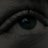# SPY label on chart?

S

##### New member
What I would like is simply a label showing me on any chart if the SPY is green or red during open session. Is this possible? Ideally, the label would read SPY and the background would be green/red.#### J007RMC

##### Active member
2019 Donor
VIP
•BenTen
S

##### New member
5 indexes
Thank you!

Is there a way to only indicate gain/loss during open session?#### BenTen

Staff
VIP
@scottrades Couldn’t you just look at the quote price or watchlist column and it will tell you exactly just that?

S

##### New member
@scottrades Couldn’t you just look at the quote price or watchlist column and it will tell you exactly just that?
My watchlist shows me at the moment if the candle is green or red, but not if its green/red on the day, in the open session. This is all I'm looking for... green/red on the day from the open.

S

##### New member
What I'm after is a label that shows me green on the day. For example, the lable in the screenshot attached should be green as spy is above its open (green line). How may I adjust this code to suit?

Code:
``````# Market Breath Ratios
# Paris (based on an earlier idea from ESP)
# 11.02.2015

def UVOL   = close(“\$UVOL”);
def DVOL   = close(“\$DVOL”);

def UVOLD  = close(“\$UVOLI”);
def DVOLD  = close(“\$DVOLI”);

def UVOLS  = close(“\$UVOLSP”);
def DVOLS  = close(“\$DVOLSP”);

def UVOLN  = close(“\$UVOL/Q”);
def DVOLN  = close(“\$DVOL/Q”);

def UVOLR  = close(“\$UVOLRL”);
def DVOLR  = close(“\$DVOLRL”);

def SPYRatio =  if (UVOLS >= DVOLS) then (UVOLS / DVOLS) else -(DVOLS / UVOLS);
AddLabel(yes, "S&P500: " + Concat(Round(SPYRatio, 2), ” :1”), if SPYRatio >= 0 then Color.UPTICK else Color.DOWNTICK);

def DJIRatio =  if (UVOLD >= DVOLD) then (UVOLD / DVOLD) else -(DVOLD / UVOLD);
AddLabel(yes, "DJI: " + Concat(Round(DJIRatio, 2), ” :1”), if DJIRatio >= 0 then Color.UPTICK else Color.DOWNTICK);

def NDXRatio =  if (UVOLN >= DVOLN) then (UVOLN / DVOLN) else -(DVOLN / UVOLN);
AddLabel(yes, "QQQ: " + Concat(Round(NDXRatio, 2), ” :1”), if NDXRatio >= 0 then Color.UPTICK else Color.DOWNTICK);

def RUTRatio =  if (UVOLR >= DVOLR) then (UVOLR / DVOLR) else -(DVOLR / UVOLR);
AddLabel(yes, "R2000: " + Concat(Round(RUTRatio, 2), ” :1”), if RUTRatio >= 0 then Color.UPTICK else Color.DOWNTICK);

def NYSERatio =  if (UVOL >= DVOL) then (UVOL / DVOL) else -(DVOL / UVOL);
AddLabel(yes, "NYSE: " + Concat(Round(NYSERatio, 2), ” :1”), if NYSERatio >= 0 then Color.UPTICK else Color.DOWNTICK);``````Last edited:
SSPY signal based off of VIX correlation? Questions 5SPY & VXX DMI Labels for ThinkorSwim Indicators 1